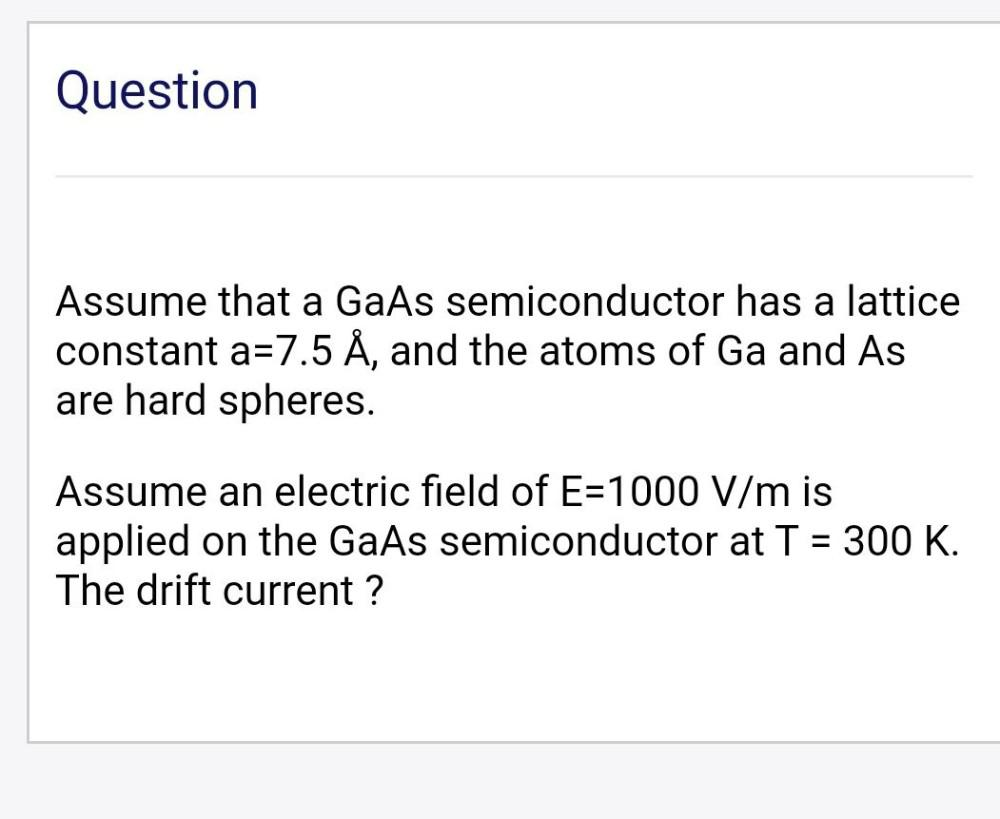Question:

# Question Assume that a GaAs semiconductor has a lattice constant a=7.5 Å, and the atoms of Ga and As are hard spheres. Assume anQuestion Assume that a GaAs semiconductor has a lattice constant a=7.5 Å, and the atoms of Ga and As are hard spheres. Assume an electric field of E=1000 V/m is applied on the GaAs semiconductor at T = 300 K. The drift current ? -